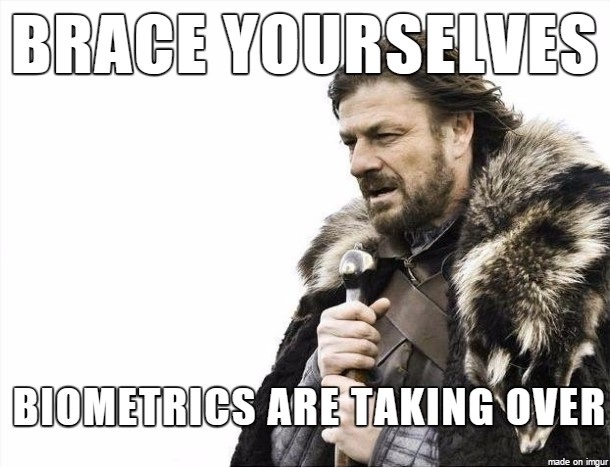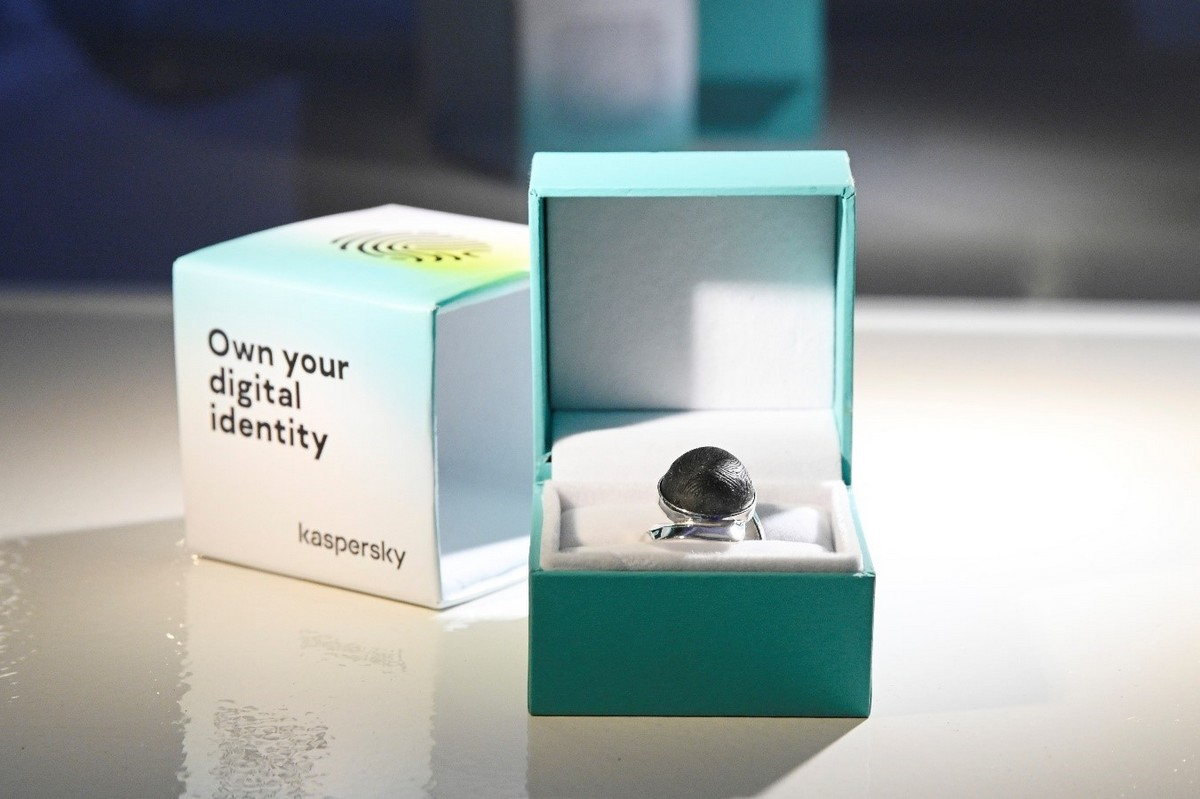# Biometrics. Lord of the ring!

It’s now perfectly normal to unlock your phone or computer with a fingerprint – nobody would bat an eyelid. In fact, more and more biometric data is being collected, whether it be facial, voice or iris recognition. This type of authentication appears to be very reliable because every human’s physical and behavioral features are unique. However, very few think of where all that data is stored and how it’s protected. What if somebody gains access to it?According to our experts, in Q3 2019 alone, 37% of computers used to store and process biometric data faced the risk of a malware infection at least once. Of these, more than 5% were infected with spyware. The main sources of infection were the internet, removable media such as flash drives, and email clients.

When your password is leaked, it’s annoying, but it’s easy to change it. But what do you do if cybercriminals get access to your fingerprints? You don’t have a spare set of fingers! We’ve given the problem some thought … and come up with a solution! :)

In early December in Milan, together with Swedish designer Benjamin Waye, we presented a unique prototype ring used for authentication.The ring is made of silver on a 3D printer, and is adorned with a specially cast “gem” of conductive rubber fibers that form a unique print with the help of specialized software. With this ring, you can unlock your phone or, say, open a smart house’s door. If the ring is lost or stolen, its print can be blocked as a method of authentication and replaced by another.

Nowhere yet, unfortunately. The ring is only a prototype and isn’t on sale. The project was meant to draw attention to the problem of protecting biometric data. But! Yours truly has gotten accustomed to playing Santa every December, so today I’m announcing a competition among the subscribers of this cozy little blog of mine. The prize? The chance to become the lord of this very ring – from a unique and very limited edition – giving you control over your biometric data.

And in order to become the proud owner of the ring, you need to solve some sort of difficult but meaningful task… What task should I set? Here we go:

You have four (and only four) digits: <1, 4, 0, 9> (just like in the number of the Moscow school where we’re involved in the Mathematical Vertical program). Armed with the mathematical knowledge from your school days, namely basic arithmetic operations (adding, subtracting, multiplying and dividing), plus raising to a power, extracting a root and factorials, you need to obtain all the numbers from 21 to 100. All four digits must be used for each number, though you can stick them together, and/or their sequence can be changed, like in the examples below for 0 – 20:

0 = 0*149
1 = 1 + 0*49
2 = 10/(9 – 4)
3 = 9/(4 – 1) + 0
4 = 4 + 0*19
5 = 10 – 9 + 4 = 14 – 9 + 0 = 4 + 1 + 0*9
6 = 9 – 4 + 1 + 0
7 = sqr(9) + 4 + 1*0 = sqr(49) + 1*0 = sqr(9) * sqr(4) + 1 + 0
8 = 9 – 1 + 4*0
9 = 9 + 0*14
10 = 9 + 1 + 0*4
11 = 10 + 4 – sqr(9)
12 = 9 – 1 + 4 + 0
13 = 9 + 4 + 0*1
14 = 14 + 0*9
15 = 10 + 9 – 4 = 19 – 4 + 0
16 = 10 + (sqr(4) * sqr(9))
17 = 9 * sqr(4) – 1 + 0 = sqr(49) + 10
18 = 9 * sqr(4) + 0*1
19 = 19 + 0*4
20 = 40 / (sqr(9) – 1) = 19 + (0*4)!

If some of the numbers cannot be obtained in this manner, then which additional operations from the high school math curriculum need to be added? (you can’t turn the numbers upside down, or round numbers up or down). Well, OK, let’s allow number’s to be turned upside down – for instance, if you obtain 66 at some stage, you can turn it round to get 99.

The first person to show how they derived all the numbers from 21 to 100 in the comments section will get the magic ring! (such a nice gift for Christmas, isn’t it?)#### Julien Ripet

(Apologies for the broken English, not my first language)
Hey, I know it’s a bit late, but I just found the post, and it took me some time to complete the puzzle. I don’t know if the competition is still running? I don’t see any other comments, but maybe others already sent their answers by PM. Anyway, it was fun to exercise my mathematics a bit, always love this kind of puzzle.

My submission :
21 : 19 + sqrt(4) + 0
22 : sqrt(4) + 19 + fact(0)
23 : 14 + 9 + 0
24 : 14 + 9 + fact(0)
25 : sqr(1 + 4) + (0 * 9)
26 : sqr(1 + 4) + fact(0 * 9)
27 : 9 * (4 – 1) + 0
28 : 4 * (9 – 1 – fact(0))
29 : fact(4) + fact(0) + 1 + sqrt(9)
30 : (4 – fact(0)) * (1 + 9)
31 : fact(4) + 9 – 1 – fact(0)
32 : fact(4) + 9 – 1 – 0
33 : fact(4) + 9 + 1 * 0
34 : fact(4) + 9 +1 + 0
35 : 4 * 9 – 1 + 0
36 : 4 * 9 + 1 * 0
37 : 4 * 9 + 1 + 0
38 : 4 * 9 + 1 + fact(0)
39 : 4 * (9 + 1) – fact(0)
40 : 4 * (9 + 1) +0
41 : 4 * (9 + 1) + fact(0)
42 : 41 + fact(0 * 9)
43 : 41 + sqrt(9) – fact(0)
44 : 41 + sqrt(9) + 0
45 : 9 * (4 + 1) + 0
46 : 9 * (4 + 1) + fact(0)
47 : 49 – 1 – fact(0)
48 : 49 – 1 +0
49 : sqr(9 – sqrt(4)) + 1 * 0
50 : sqr(9 – sqrt(4)) + 1 + 0
51 : sqr(9 – sqrt(4)) + 1 +fact(0)
52 : (9 + 4) * sqr(1 + fact(0))
53 : 9 * fact(4 – 1) – fact(0)
54 : 9 * fact(4 – 1) + 0
55 : 9 * fact(4 – 1) + fact(0)
56 : (sqr(9) – fact(4)) – 1 +0
57 : 19 * (4 – fact(0))
58 : (sqr(9) – fact(4)) + 1 + 0
59 : (sqr(9) – fact(4)) + 1 + fact(0)
60 : (1 + 9) * fact( 4 – fact(0))
61 : sqr(9 – 1) – sqrt(4) – fact(0)
62 : sqr(9 – 1) – sqrt(4) – 0
63 : sqr(9 – 1) – fact(0 * 4)
64 : sqr(9 – 1) – 4 * 0
65 : sqr(9 – 1) + fact(0 * 4)
66 : sqr(9 – 1) + sqrt(4) + 0
67 : sqr(9 – 1) + sqrt(4) + fact(0)
68 : sqr(9 – 1) + 4 + 0
69 : sqr(9 – 1) + 4 + fact(0)
70 : ( 9 – sqrt(4)) * 10
71 : fact(4) * sqrt(9) – 1 +0
72 : 9 * (4 * (1 + fact(0)))
73 : fact(4) * sqrt(9) + 1 + 0
74 : sqr(9) – fact(4 – 1) – fact(0)
75 : sqr(9) – fact(4 – 1) + 0
76 : sqr(9) – 4 – 1 + 0
77 : sqr(9) – 4 + 1 * 0
78 : sqr(9) – 4 + 1 + 0
79 : sqr(9) – 4 + 1 + fact(0)
80 : sqr(9) – 1 + 0 * 4
81 : sqr(9) + 0 * 1 * 4
82 : sqr(9) + 1 + 0 * 4
83 : sqr(9) + sqrt(4) + 0 * 1
84 : sqr(9) + sqrt(4) + 1 + 0
85 : sqr(9) + 4 – 1 + 0
86 : sqr(9) + 4 + 1 * 0
87 : sqr(9) + 4 + 1 + 0
88 : 91 – 4 + fact(0)
89 : 91 – sqrt(4) + 0
90 : 91 – sqrt(4) + fact(0)
91 : 91 – 4 * 0
92 : 91 + fact(4 * 0)
93 : 94 – 1 + 0
94 : 94 + 0 * 1
95 : 94 + 1 +0
96 : sqr(9 + 1) – 4 – 0
97 : sqr(9 + 1) – sqrt(4) – fact(0)
98 : sqr(9 + 1) – sqrt(4) – 0
99 : sqr(9 + 1) – fact(4 * 0)
100 : sqr(9 + 1) – 4 * 0

So there you go !
I didn’t turn numbers round, as I wasn’t sure what would be allowed ( 5’s into 2’s and such). I think they are all correct, but if there’s any mistakes, let me know.

Thanks again for the puzzle, and happy new year ;)

Leave a note